## plot of fracdiff of f(x)=x...

Why doesn't the graphs of alpha=0 and alpha=1  appear in the following code?

```restart:
v:=t->t:
plot([seq(fracdiff(v(t), t,alpha),alpha=-1..1.5,0.5)],t=0..10,view=[0..9.5,0..5],legend=[seq('alpha'=alpha,alpha=-1..1.5,0.5)],color = [red, blue, green,yellow,cyan,magenta],axis=[gridlines=[linestyle=dot]]);
```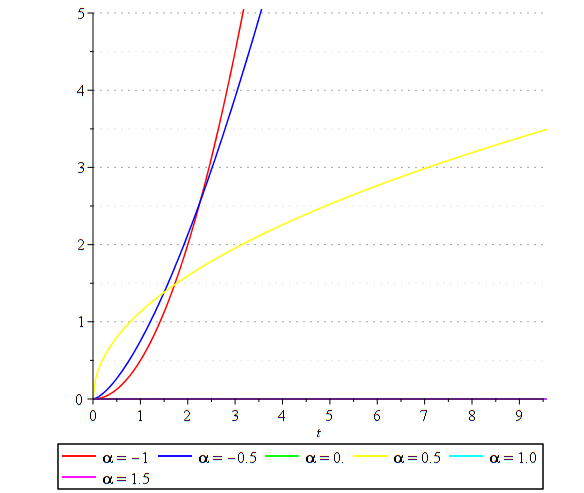if alpha=1;

`fracdiff(t, t,alpha)`

gives 1. But it doesn't appear in the figure.

if alpha=0,

`fracdiff(t, t,alpha)`

gives t. But it doesn't appear in the figure, also.

## How can I remove a section without removing its co...

How can i remove a section envelope ( the initial arrow and the vertical line) without removing the content.

In other words how can I liberate the content from being imprisoned in the section

Thank you

## Animation function not working...

Hi,

I would like to create an animation of the solutions to a differential equation, but I can't get animation to work.  I copy and pasted

plots[animate]( plot, [A*sin(x), x=0..10], A=0..2 )

into maple, but the animation did not animate, and only plotted the result for A = 0.  I am using Maple 2019.  Any ideas?

## Code Snippets or File Content?...

Hi,
I see more and more contributions where the content of the mw file is replaced by a code snippet and, possibly, an image as illustration.
Do we have to consider this as the new norm to post question and to submit replies or answers?

(this question is related to the one Carl Love posted a few days ago  Why can't I display any worksheet? and which, if I'm not mistaken, has not been definively answered)

## How to plot IF statment?...

restart:
nuhf:=0.294*10^(-6):
Rehf:=Vhf*H/nuhf:
Vhf:=0.5:
rhohf:=965.31:
Lhs:=2.5*10^(-3):
DP1:=f1*Lhs*rhohf*Vhf^2/(2*H):
f1:=4*18.3/Rehf:
Whs:=1:
mhf:=rhohf*Vhf*Whs*H:
Rehf:=Vhf*H/nuhf:
PL1:=DP1*mhf/rhohf:
DP2:=f2*Lhs*rhohf*Vhf^2/(2*H):
f2:=0.3164/(Rehf^(0.25)):
PL2:=DP2*mhf/rhohf:
procd:=proc(H)
if Rehf<1000 then PL1 elif Rehf>1000 then PL2 else 0 fi;
end;

plot('procd(H)',H=0..0.002);

## what does it mean when solve returns just the vari...

When doing solve(res,x); maple returns back x

Does this means the equation is true for any x?

Also, doing PDEtools:-Solve(res,x) returns nothing.

I was trying to check if my solution is valid for an ode, and I obtained res above is what odetest() gives back, I wanted to find for what could res be zero.

Here is the code

```restart;
ode:=diff(y(x),x)=2*sqrt(1+y(x))*cos(x);
my_sol:=y(x)=sin(x)^2+2*sin(x);
res:=odetest(my_sol,ode);
res:=simplify(res)=0;
solve(res,x);

```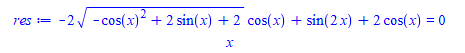And now

```PDEtools:-Solve(res,x)
```

gives nothing.

So I am a little confused what is going on. does solve result mean the equation is true for any (I think the solution I have is correct, so may be what this means,  but wanted to make sure, as I do not think I've seen this before)  And if so, why then PDEtools:-Solve did not return the same result.

Maple 2020.2, windows 10, Physics 897

## Can I redirect Maple output that includes tables ...

I know that I can use writeto() and appendto() to redirect Maple output to a file.  However, I have not been able to get this to work properly with tables or special characters.

Is there a way to change this?

## Why the figure is rougher in implicitplot when I i...I'm new to Maple, recently I'm focusing on the implicitplot.  "Help" tells me that gridrefine specifies the number of recursive subdivisions to perform on any cells in the initial grid that have been determined to contain part of the curve to be plotted. Now I have a function to implicitplot. I find that when I increase the gridrefine, the figure is rougher, why does this happen since the recursive subdivisions are increased?

restart: F:=(x,y) -> -(1/2)*(25*(-2/25+y^2*(x^2+1)))*(1/2)^((-x^2+1)/(x^2+1))+4*(1/2)^(2*x^2/(x^2+1))*(1/25)+5*y^2*(1/2)^(-2*x^2/(x^2+1)); Digits:=20; plots:-implicitplot(F,0..20,0..10,gridrefine=3,crossingrefine=5,numpoints=1000,labels=["x","y"]); plots:-implicitplot(F,0..20,0..10,gridrefine=6,crossingrefine=5,numpoints=1000,labels=["x","y"]);

## question on RootOf...

Maple gives solution to equation as RootOf when there is no explicit solution

```restart;
eq:=x*sin(x*y)+y+1=0;
sol:=solve(eq,y)
```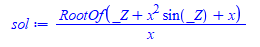The _Z above, is the variable we wanted to solve for, which is in this example. My question is, why did not Maple give the solution as

```sol2:=RootOf(x*sin(_Z*x)+_Z+1)
```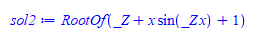Which is a little simpler looking. Is the above not the same as what Maple gives?  I just replace by _Z

```Digits:=100;
plot(evalf(sol-sol2),x=0..Pi)```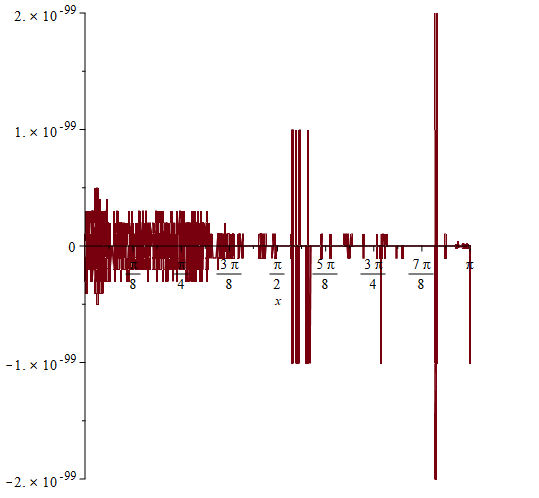## Is there a general parametric definition of a peda...

The uploaded worksheet contains the general definition of a pedal curve related to the curve [f(t),g(t)], and includes several example pedal curves.

Is there a comparable general definition of a pedal surface? If so, in your answer please include the Maple code for one or two simple examples e.g. relating to a sphere, to an ellipsoid.

To everyone in the Maple Forum community, Happy Holidays and a fulfilling New Year!

## How to manage homogenous coordinates...

If the equation with homogeous coordinates in the base (A,B,C) is pYZ+qZX+rXY=0, an affine  equation in the base (A,AB,AC) is
pxy+qy(1-x-y)+r(1-x-y)=0, it is also written rx²+(q+r-p)xy+qy²=0. The discrim of  trinom is rx²+(q+r-p)xy+qy² is of the sign of
delta=(q+r-p)²-4qr; We get a hyperbole, a parabole, an ellipse according to delta is >0, =0 or <0 respectvely. How implement
this property. Thank you very much.

## How to eval integration under loop...

Here is my try to integrate the expression L with trapozoid or simpson

numerical_int.mw

## plot options for Volume Of revolution...

Hi,

Just to inform me about the options to add in my code , to have a style similar to the  picture..

Thanks

QuestionOptionVolume.mw## Why does dsolve give an incorrect solution?...

Why do I get this wrong result when I try to solve formally this ode (note that acer has already obtained its implicit form here implicit)

```restart:
interface(version)
Standard Worksheet Interface, Maple 2015.2, Mac OS X, December 21 2015 Build ID 1097895

edo := diff(y(x), x, x) = 1/y(x) - x*diff(y(x), x)/y(x)^2;
ic  := y(0)=1, D(y)(0)=0:

dsolve({edo, ic}, y(x));
eval(edo, %)
/ d      \
x |--- y(x)|
d  / d      \    1       \ dx     /
--- |--- y(x)| = ---- - ------------
dx \ dx     /   y(x)          2
y(x)

y(x) = 1

0 = 1```

## Finding when odetest is zero when csgn appears in ...

sometimes Maple's solution for an ode is not valid for all x. When I run odetest on the solution, it is not zero.

For the case when the output of odetest contains one csgn(), I am trying to determine if odetest result will become zero, when csgn is +1  or -1. If so, then next look into the argument of csgn and solve for x under this condition and hence find the range of x when the solution is valid.

I wrote the following to do this and check the idea.

I'd like to ask if the Maple experts here can suggest improvement or if there a better way to do this.

The code below works if there is only instance of csgn() in the output of odetest. Which covers almost all the cases I saw so far.

The code first checks if csgn is present in the output of odetest. If so, it then uses indents to pick up the function csgn out. Then uses op to pick its argument. Then uses solve with inquality to find when the argument is either positive or negative. This results in the range of x needed to make odetest zero.

This is just a quick prototype to test the idea. This not the final code I will be using as that will include more checks and be more robust.

```restart;
ode :=diff(y(x),x)=2*(x*sqrt(y(x))-1)*y(x):
ic  :=y(0)=1:
sol :=dsolve([ode,ic]):
res :=odetest(sol,ode);```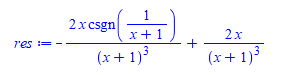So we see odetest did not give zero.  The issue is that I can't just do

`solve(res=0,x)`

It does not work.  So that is why I had to look inside as follows:

The goal is to check if when csgn is either +1 or -1, if it will then become zero.

And if so, then also find the range of x which will caused this.  In the above, we see that when 1/(x+1) is +1, then odetest result  will become zero. This means x has to be larger than -1.

Note that this is all done in code, without being able to look at the screen and then decide what to do

```if res<>0 then
if has(res,csgn) then
if simplify( subsindets(res,csgn(anything),f->1)) = 0 then
print("the odetest becomes zero when csgn is POSITIVE");
Z:=indets(res,specfunc(csgn));
the_args:=map(x->op(x),Z);
print("Now solve ",the_args," for x, when the expression is POSTIVE");
for tmp in the_args do
result:=solve(tmp>0,x);
print("the solution is valid for x>", op([1,1],result));
od;
else
if simplify( subsindets(res,csgn(anything),f->-1)) = 0 then
print("the odetest becomes zero when csgn is POSITIVE");
indets(res,specfunc(csgn));
the_args:=map(x->op(x),Z);
print("Now solve ",the_args," for x, when the expression is negative");
for tmp in the_args do
result:=solve(tmp<0,x);
print("the solution is valid for x<", op([1,1],result));
od;
else
print("give up. Tried when csgn is positive or negative");
fi;
fi;
fi;
fi;```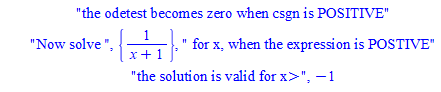If there is more than one csgn function in the odetest result, it will become much more complicated, since one have to check all combinations to find under which combination odetest will become zero. So for now, I am just doing this only for one case of csgn present.

﻿